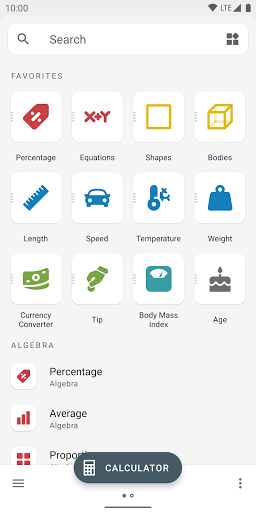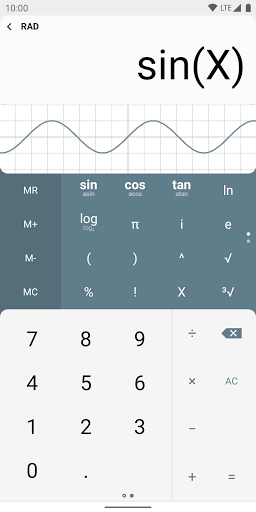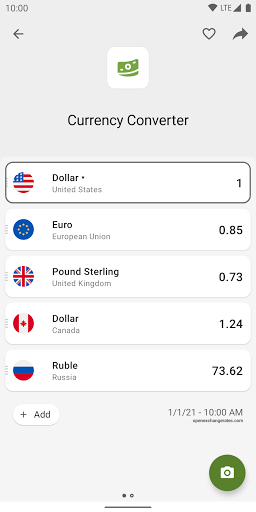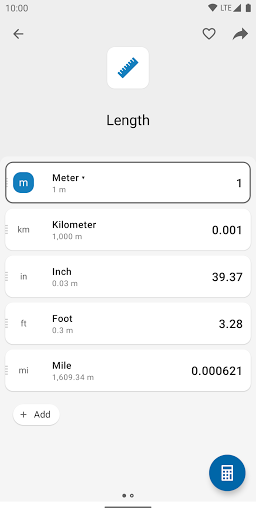# All-In-One Calculator and Unit Converter Pro v4 Paid APK

Updated on

## App Screenshots# All-In-One Calculator and Unit Converter Pro v4 Paid APK

Requirements: Android 4.0.3+
Overview: All-in-one Calculator and Unit Converter is an easy to use app with features that you need.

This app included 15+ Unit Converters, Ideal Body Weight Calculator, Tip Calculator and many other features that you will love.

#### All-In-One Calculator and Unit Converter Features

• General Calculator – Supports general mathematical operations such as addition, subtraction, multiplication, division, square root, and power
• Algebra Calculator – Supports Equation Solver, Fractions, GCF / LCM, Percentage
• Unit Converter – Supports conversion of units of Angle, Area, Data Storage, Data Transfer, Energy, Length, Number System, Pressure, Shoe Size, Speed, Temperature, Time, Volume, Weight
• Health Calculators – Supports Basal Metabolic Rate (BMR), Body Mass Index (BMI), Body Fat Percentage, Calorie Intake, Ideal Body Weight
• Finance Calculators – Supports Compound Interest, Discount, Simple Interest, Tip Calculator
• Geometry Calculators – Supports Perimeter, Area, Volume, Surface Area Calculators along with Heron’s Formula Calculator
• Time Calculators – Age Calculator, Elapsed Time Calculator (for both dates and times)

#### MOD Features

• Pro Unlocked.

0/5
Rate this post